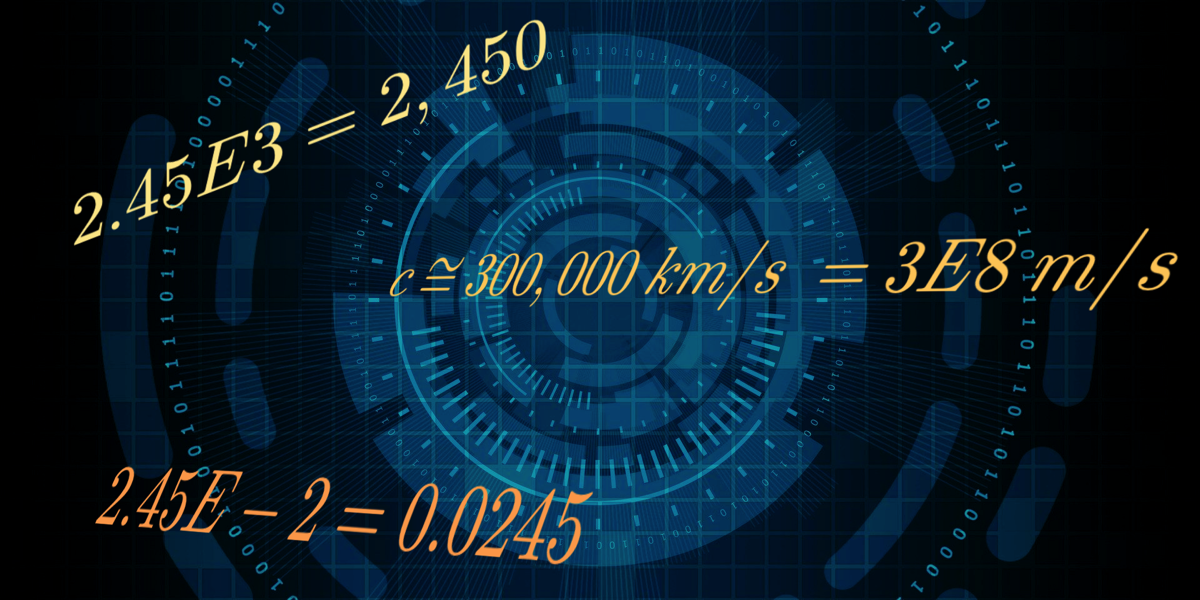# The Blog of Mathedu Academy

### A content for the curious of the mathematics in the real world… and beyond# The Exponential Notation for the Floating Real Numbers

Our scientific calculators deal sometimes with numbers with a $$E$$ inside: this is the so called Exponential, or Scientific Notation.

But what does that mean, and how to read such numbers?

These numbers have something to do with the (positive, negative or zero) powers of $$10$$.

The positive powers of $$10$$ are defined as: $$10^k$$ is $$10$$ multiplied by itself $$k$$ times:
• $$10^1 = 10$$
• $$10^2 = 10 \times 10 = 100$$
• $$10^3 = 10 \times 10 \times 10= 100 \times 10 = 1,000$$
• $$10^{k+1} = 10^k \times 10 =$$ a one with $$(k+1)$$ zeros.
The negative powers of $$10$$ are defined as: $$10^{-k}$$ is the inverse of $$10^k$$:
• $$10^{-1} = \frac{1}{10} = 0.1$$
• $$10^{-2} = \frac{1}{100} = 0.01$$
• $$10^{-3} = \frac{1}{1,000} = 0.001$$
• $$10^{-k} = \frac{1}{10^k} =$$ a zero, followed by a dot, then $$(k-1)$$ zeros and a one.
A consistent convention for ten to the power zero is:
• $$10^0 = 1$$

But what is the link between these powers of $$10$$ and the exponential notation? It is because the scientific notation is a simple way to represent powers of $$10$$:
• $$10^k = 1Ek$$
• $$10^{-k} = 1E{-k}$$
• $$1=10^0=1E0$$

And as a consequence, it is a way to represent very big numbers, as well as the tiniest ones, the closest to $$0$$.

But to do that, we must return to the powers of $$10$$, and their multiplicative properties:
• multiply by $$10^k$$ an integer is adding $$k$$ zeros to the right of that number: $$13 \times 10^3 = 13,000$$
• multiply by $$10^k$$ a decimal number is shifting the decimal point by $$k$$ places to the right, filling with zeros if needed to the right of the last digit: $$2.45 \times 10^2 = 245, \; 2.45 \times 10^4 = 24,500$$.
• multiply by $$10^{-k}$$ a decimal number is shifting the decimal point by (\ k \) places to the left, adding a zero before the decimal point if needed, and filling by zeros if needed the places between the decimal point: $$24.5 \times 10^{-1} = 2.45, \; 24.5 \times 10^{-2} = 0.245, \; 24.5 \times 10^{-3} = 0.0245$$.
• to multiply by $$10^{-k}$$ an integer, just add a “$$.0$$” at the end of to to transform it into a decimal number, then proceed like with the decimal numbers, ending in suppressing the final zero: $$24 \times 10{-1} = 24.0 \times 10{-1} = 2.40 = 2.4$$.

Now we are ready for the exponential notation. All we have to do is to replace the “$$\times 10^b$$" ($$b$$ signed integer) by "$$E \; b$$, so that $$aEb$$ is read “$$a, 10$$ to the power $$b$$“ : $$2.45 E {+3} = 2,450, \; 2.45 E {-3} = 0.0045$$.

The only last thing you need to do is that the number $$a$$ before the “$$E$$“ is called the mantissa, that it is conventionally with only one digit before the decimal point, and that that digit is non-zero.

Doing so allows you to assess that $$1 < a < 10$$, so that, multiplying the three members of the double inequality by $$10^b$$,:$$10^b < aEb < 10^{b+1}$$, so that the "exponent" $$b$$ is the order of magnitude of any $$aEb$$, and ranks the real numbers in increasing order.

More precisely, the bigger the exponent $$b$$ is in the positive domain, the much bigger the numbers $$aEb$$ are, with a factor $$10$$ gained on the interval at each step of $$1$$ of $$b$$.

For instance, the approximated light speed of $$300,000 \; km/s$$ is written in scientific notation $$3E{+5} \; km/s$$, and is of the order of magnitude of the hundred thousands; i.e $$10^5$$.

In the international metric system, it is given in $$m/s$$, which needs to multiply the $$km/s$$ by $$1,000 = 10^3$$, and thus to add $$3$$ to the exponent, so that it is approximately $$3 E{+8} \; m/s$$.

And the most negative the exponent $$b$$ is , the tiniest the numbers $$aEb$$ are, with a division by $$10$$ of the interval at each step of $$-1$$ on the exponent.

For instance, the weight of a molecule is in microgram, the millionth part of the gram, so that a molecule of $$2 \mu g$$ weights $$2 E{-6}$$, and its weight in grams is of order one millionth, or $$10^{-6}$$.
Back